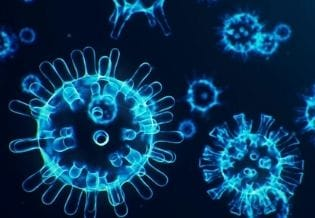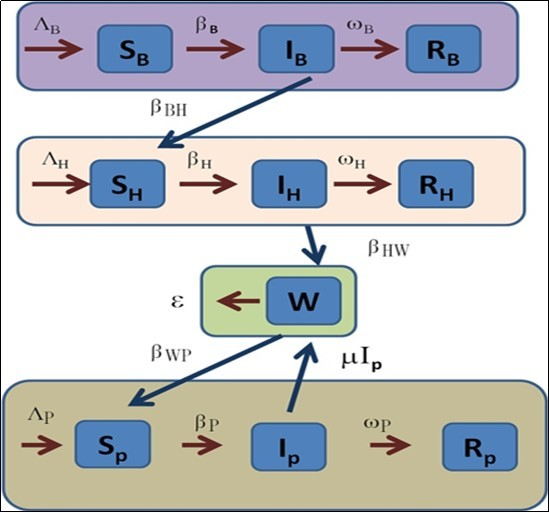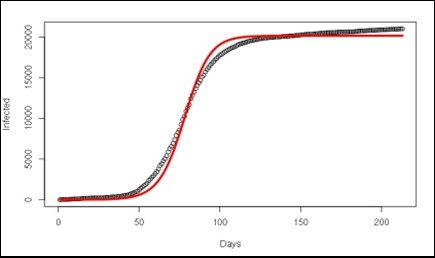### International Journal of Coronaviruses

Current Issue Volume No: 2 Issue No: 4

Research Article Open Access Available online freely Peer Reviewed Citation

# Analytical Solutions of the Transmissibility of the SARS-CoV-2 in Three Interactive Populations

### Raúl Isea 1

1Fundación IDEA, Hoyo de la Puerta, Baruta, Venezuela.

## Abstract

This paper resolves analytically a mathematical model that reproduces the transmission of Covid-19 in three interactive populations, i.e. from the initial source of contagion associated with the bat population, subsequently transmitted to unknown host (usually associate with pangolins). The host were sent and distributed to Seafood Market in Wuhan (defined reservoir), and finally infected to the human population. The model is based on a system of ten differential equations reproducing all the possible infection scenarios among all of them, that is: (1) there is no infection in any of the three populations, (2) only the population of bats is infected, (3) only the pangolins, (4) only the human people. Later, combinations between them, this is: (5) both the bat and pangolin populations, (6) bats and humans, (7) pangolins and humans, and finally, (8) all the previous populations. In each scenario, I deduced the critical points as well as the eigenvalues ​​that indicate the equilibrium conditions. Finally, it is demonstrated the validity of the model with the data corresponding to the second wave of infections in Australia.

##### Author Contributions
Received 28 Jan 2021; Accepted 28 Jan 2021; Published 02 Feb 2021;

Academic Editor: Jose Luis Turabian, Health Center Santa Maria de Benquerencia Toledo, Spain.

Checked for plagiarism: Yes

Review by: Single-blindThis is an open-access article distributed under the terms of the Creative Commons Attribution License, which permits unrestricted use, distribution, and reproduction in any medium, provided the original author and source are credited.

Competing interests

The authors have declared that no competing interests exist.

Citation:

Raúl Isea (2021) Analytical Solutions of the Transmissibility of the SARS-CoV-2 in Three Interactive Populations. International Journal of Coronaviruses - 2(4):1-8. https://doi.org/10.14302/issn.2692-1537.ijcv-21-3732

## Introduction

The World Health Organization declared a new pandemic caused by a virus called as Severe Acute Respiratory Syndrome Coronavirus 2 (SARS-CoV-2) on March 11, 2020, after the first case detected in the Seafood Market of Wuhan province in China in December 2019. Until January 02, 2021, a little more than 84 million confirmed infections and approximately two million deaths had been reported.

Up-to-date, there is a wide range of mathematical models that explain the spread of the virus, but most of all are based on contagion in the human population 1, 2, 3, 4, 5. However, when reviewing the scientific literature, there is a only paper that propose a model to explain the transmissibility and dynamic of the transmission of the virus based on the Bats-Hosts-Reservoir-People model 6. This paper only determined an only equilibrium condition corresponding to the trivial solution of the system. This model is based on the transmission of the virus between three different populations, it means, from the source of contagion in humans originated in bats 7, later transmitted to an unknown host. As indicated Chen et al., the host were hunted and distributed in the Seafood Marked in Wuhan (defined as Reservoir), and finally infected to the human population.

For this reason, the objective of this paper is to propose a mathematical model that is capable of reproducing all the possible scenarios of virus contagion in the transmission of the virus from the bat population to the human infection.

## Mathematical Model

The model is inspired in the paper published by Chen et al.6, where they proposed a deterministic compartmental model composed of fourteen differential equations. Based on this paper, I present a new version of this model, but based on SIR-type compartment model as shown as in Figure 1.

Figure 1.The Flowchart of the model proposed in this paper. The infection source is shown in violet color and it is a bats population, represented with B subscript. The host region is pink and depicted with the H subscript (some bioinformatics studies indicated that could be pangolin population 8). The Reservoir (green region) was the Seafood Market in Wuhan, and now is any region in the world. Finally, the human people are the last section and identified with the P subscript.This new model is based on the following suppositions:

The populations susceptible to contracting Covid-19 are SB, SH and SP corresponding to the bat, pangolin and human populations, respectively. They are the healthy population who have not yet infected.

ΛB, ΛH and ΛP son the newborn bats, pangolins and human, respectively. These values ​​should be obtained by fitting the model with the collected data.

The contagion rate in bats, pangolins and humans is given by the constants βB, βH, and βP, respectively. These values should be obtained by fitting the model with the collected data.

mB, mH and mP represent the death rate of bats, pangolins and human, respectively. In the case of the human population, mP is equal to 0.0000456.

NB, NH, and NP are the total populations of bats, pangolins and human, respectively.

Populations infected by the virus are represented by IB, IH and IP corresponding to the bat, pangolin and human populations, respectively.

ωB, ωH and ωP are the infectious period of bats, pangolins and human, respectively. These values should be obtained by fitting the model with the collected data.

The reservoir is denoted as W.

RB, RH and RP represent the recovered bat, pangolin, and human populations, respectively.

The infection rate between bats and pangolins is given by the variable βBH, where the superscript and subscript identify the beginning and end of the rate of infection, respectively; while the contagion rate from pangolins to the reservoir is given by βHW, and βWP is from reservoir to human. These values ​​should be obtained by fitting the model with the collected data.

ε is the lifetime of the virus in the Reservoir. Given that each country maintains different policies to combat the virus, and for this reason, it is necessary to fit according to infected data.

µ is the transmission period from IP to W.

The system of differential equations proposed in this paper is the following:

Finally, indicate that there are three major differences in the model developed in this work with respect to the one proposed by Chen et al.6. First, the Chen et al. model is SEIR-type model, and here it is of a SIR-type one. Second, Chen et al. divided the human population into five compartments (Susceptible, Exposed, Infected, Asymptomatic, and Recovered), while this work is divides in three (Susceptible, Infected and Recovered). Finally, Chen et al. only propose the trivial solution of the system, ie., when there are no sources of infection; while the present work resolves all possible scenarios where Covid-19 can be transmitted.

## Results and Discussion

The analytical resolution of the system of equations is based on the same procedure used and validated in a wide range of scientific works 1, 2, 3, 4, 5, so only the results will be shown in this paper. They are found eight different scenarios represented as (PCi, i = 1 to 8).

## First Critical Point

The first critical point (PC1) is the trivial solution of the system, that is, when there are no infections in any of the populations:

This result indicates the population that may be susceptible to contracting the virus are equal to ΛBB, ΛH/mH and ΛP/mP and corresponding to the populations of bats, pangolins and humans, respectively.

The next step is to determine the equilibrium conditions, it means, the result of evaluating the Jacobian of the system in this critical point. By doing this, three different eigenvalues are obtained:

where three new variables (ΔH, ΔB, and ΔP) have been introduced indicated in (Table 1) to simplify the equation in this paper. These solutions reveal that the different populations depend exclusively on each population and are not conditioned on each other.

Table 1. Definitions made to simplify mathematical expressions obtained at work

## Second Critical Point

The second critical point (pc2) is when only the bat population is infected by Covid-19, and the susceptible population of pangolins can infected by the virus, without them being able to infect humans:

This result reveals that the population of pangolins that can be infected directly proportional to the rate of infection between bats and pangolins. By repeating the procedure described above, the eigenvalues are

The first eigenvalue indicates the equilibrium condition in the bat population, while the third corresponds to the human population (which should be the same as the previous scenario). Finally, the second eigenvalue indicates the condition when the parameters that relate the contagion rate between bats and pangolins are combined.

## Third Critical Point

The third critical point (pc2) relates to the scenario when the pangolin population (host) is infected:

It should be noted that the human population that can be infected by the virus depends directly on the rate of infection with the environment (Reservoir). So it is necessary to control and reduce the presence of the virus in the environment in order to eradicate the chain of infections in the human population.

The eigenvalues obtained are:

The second eigenvalue is the one that gives us new information as it is a combination of conditions between the pangolin and human populations.

## Fourth Critical Point.

The fourth critical point (pc4) represents the solution of the human population when it is infected by Covid-19:

Where the new variables indicated in this critical point are listed in Table 1. The three eigenvalues in this scenario are:

The third eigenvalue is a mixture of the different parameters corresponding to human and pangolin populations.

## Fifth Critical Point

The fifth critical point (pc5) corresponds to the case when the population of bats and pangolins are infected by Covid-19, so that the virus is also present in the Reservoir, and the human susceptible population can transmit the disease without being infected, that is:

This solution reveals how the environment (W*) increases the number of people susceptible to contracting the disease. In this case, only two eigenvalues were determined:

As can be seen above, the first eigenvalue depends exclusively on the parameters of the bats, while the second is a combination of the parameters between the three populations.

## Sixth Critical Point

The sixth critical point (pc6) corresponds to the case when bat populations and humans are infected, that is:

The eigenvalues are:

The first two eigenvalues depend on the parameters of the bats and the human population, respectively; while the latter is a combination between the parameters between the populations of pangolins and bats.

## Seventh Critical Point

The seventh critical point (pc7) corresponds to the case when pangolin populations and humans are infected:

The human population that is susceptible to contracting the virus increases over time. In this case, only two expressions of the eigenvalues can be obtained:

that is, the eigenvalues corresponding to the bat population.

## Last Critical Point

The last critical point (pc8) corresponds to the case when the three populations are infected by Covid-19:

This critical point is when everyone is infected, and unfortunately it has not been possible to determine the eigenvalues for this system, in view of the complexity of the equations.

## Numerical Example

The main advantage of this mathematical model is that each scenario can be considered separately. As an example, the Figure 2 shows the result of the least squares fitting of the observed contagion data registered in Australia, but due to limitations of the computational equipment, only the cases that occurred from 2020/06/03 to 2021/01/02 are analyzed (corresponding to the second wave of contagions). Here, the different parameters corresponding to the populations of bats and pangolins were considered equal to zero.

Figure 2.The least squares fitting (red color) and the observed contagion data (black) in Australia.The observed contagion data was obtained from John Hopkins University. The values ​​obtained by least squares were: βWP = 1.708, βp= 1.215, ωP = 0.001, ΛP = 81.212, µ = 1.100, ε = 0.001, (setting the mP value to 0.0000456). According to these results, the population susceptible to contracting the virus in Australia is equal to 1,780,965 (maximum value).

## Conclusions

This is the first mathematical model that studied the different transmission scenarios of Covid-19 in three different populations, it means, bats, pangolins and humans. The model is based on a compartmental scheme of the SIR-type (Susceptible-Infected-Recovered), and despite the simplicity of the model, eight critical points are obtained representing the different infection scenarios among the three populations considered in this paper. In the next publication, the model will be analyzed considering the measures of social distancing as well as the effectiveness of vaccines in various countries that are infected by Covid-19.

## Acknowledgments

The author appreciates the unconditional help of Rafael Mayo-Garcia when reading this work immersed in a sea of differential equations. Finally, thank God for his continuous inspiration in the development of this manuscript.